The Gyro Sensor

The Gyroscopic Sensor is typically used to measure rotational speed, but it can also calculate rotational change. When your robot turns around, the Gyro Sensor can measure the robot's turn rate, in degrees per seconds. This rotational speed is measured at a rate of 1000 samples per second. Therefore, by multiplying the speed by the duration of the turn, the gyro sensor can also tell how much the robot has turned, in degrees.

This is incredibly useful when trying to perform accurate turns to the degree, such as a 90 degree turn or a 180 degree turn.

When starting a program, the Gyro Sensor's starting value is 0 degrees. It then adds or subtracts the amount of degrees the robot has turned. This means that the Gyro Sensor sometimes shows negative values!

Legacy Cyber Robotics 101 (Desert theme; large HUD)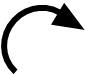When EV3 Robot turns right (clockwise) the gyro readings will go down.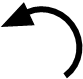When turning left (counterclockwise) the readings will go up.

Here's what it looks like when EV3 Robot turns 90 degrees to the right (to -90), then back to 0 (facing front), then 90 degrees to the left (to 90), then finally back to 0 degrees: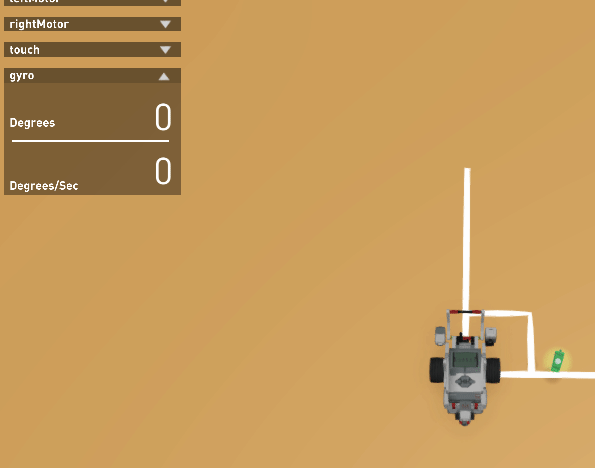New Cyber Robotics 101 (Farm theme; small HUD)When EV3 turns right (clockwise) the Y axis gyro readings will go up.When turning left (counterclockwise) the readings will go down.

Here's what it looks like when EV3  turns 90 degrees to the right (to 90), then back to 0 (facing front), then 90 degrees to the left (to -90), and back to 0 degrees: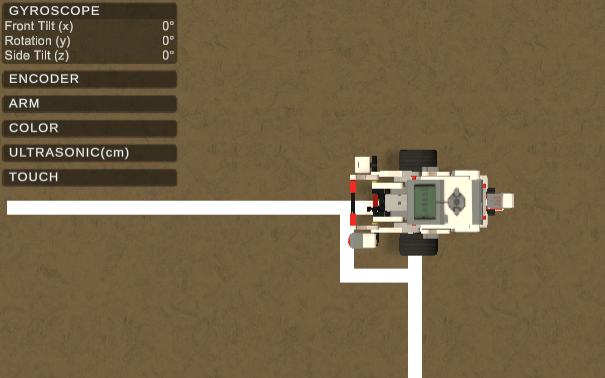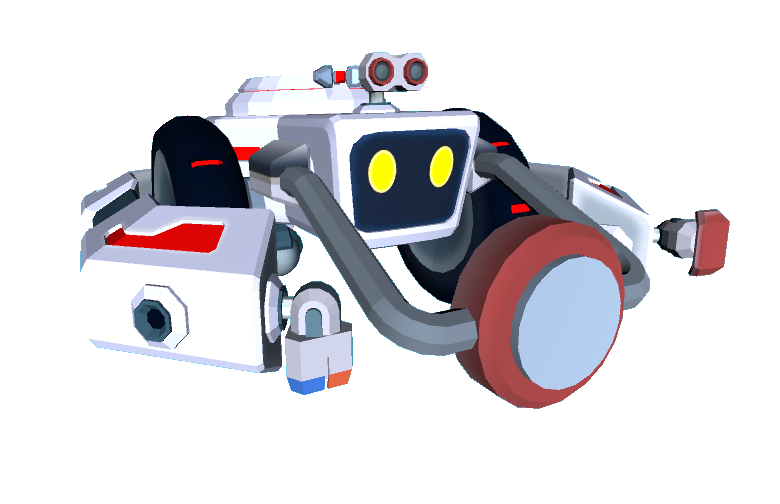Ruby RobotWhen Ruby turns right (clockwise) the Y axis gyro readings will go up.When turning left (counterclockwise) the readings will go down.

Here's what it looks like when Ruby Robot turns 90 degrees to the right (to 90), then back to 0 (facing front), then 90 degrees to the left (to -90), and back to 0 degrees: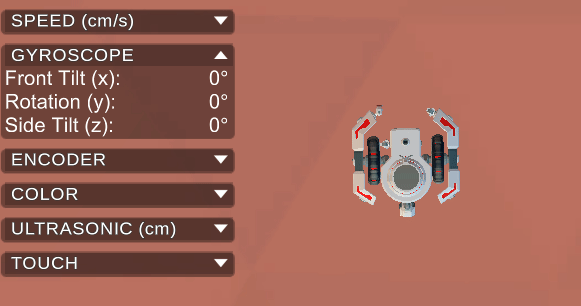Note that the faster you turn, the harder it is for the Gyro Sensor to keep up! Therefore, accurate turns require either low speeds or some level of speed control.

The Gyro Sensor can easily be reset to show 0 degrees, allowing you to start counting degrees from the robot's position even after it has made several turns.

Get Gyro Reset Block (EV3 and Ruby)

Wait Until Gyro (EV3)

Get Gyro Axis Block (Ruby)

Get Gyro Y Axis Block (Ruby)